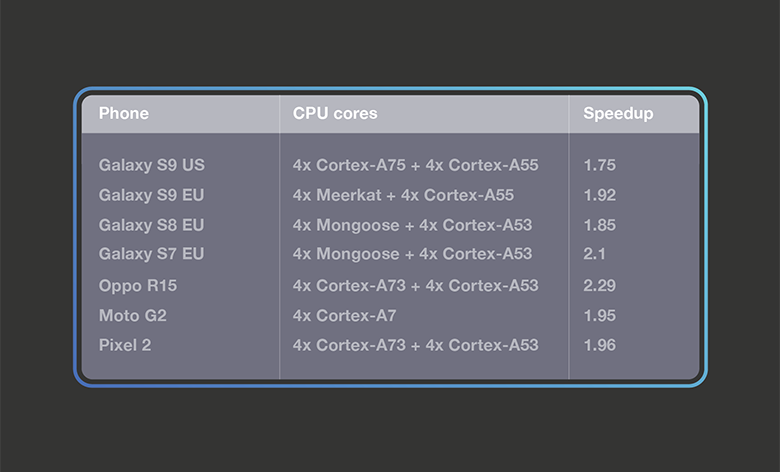• Facebook is open-sourcing QNNPACK, a high-performance kernel library that is optimized for mobile AI.
• The library speeds up many operations, such as depthwise convolutions, that advanced neural network architectures use.
• QNNPACK has been integrated into Facebook apps, deployed to billions of devices.
• On benchmarks such as quantized MobileNetV2, QNNPACK outperforms state-of-the-art implementations by approximately 2x on a variety of phones.

To bring the latest computer vision models to mobile devices, we’ve developed QNNPACK, a new library of functions optimized for the low-intensity convolutions used in state-of-the-art neural networks.

QNNPACK, which stands for Quantized Neural Network PACKage, is integrated into the Facebook family of apps and has been deployed on more than a billion mobile devices globally. With this new library, we can perform advanced computer vision tasks, such as running Mask R-CNN and DensePose on phones in real time or performing image classification in less than 100ms even on less-powerful mobile devices.

We are open-sourcing QNNPACK to provide comprehensive support for quantized inference as part of the PyTorch 1.0 platform. QNNPACK is immediately usable via Caffe2 model representation, and we are developing utilities to export models from PyTorch’s Python front end to the graph representation. We’re also working on optimizations for these operations on other platforms, beyond mobile.

Because mobile phones are 10x-1000x less powerful than data center servers, running state-of-the-art artificial intelligence requires several adaptations to squeeze all available performance from the hardware. QNNPACK delivers this by providing high-performance implementations of convolutional, deconvolutional, and fully connected operations on quantized tensors. Before QNNPACK, there wasn’t a performant open source implementation for several common neural network primitives (grouped convolution, dilated convolution); as a result, promising research models such as ResNeXt, CondenseNet, and ShuffleNet were underused.

## New optimization needs for cutting-edge AI on mobile

When Facebook started deploying neural networks to phones two years ago, most architectures for computer vision were built upon convolutional operations with large kernels. Such operations are notorious for high computational intensity: Direct implementation involves many multiply-add operations per every loaded element. Caffe2Go uses a kernel library called NNPACK — which implements asymptotically fast convolution algorithms, based on either Winograd transform or Fast Fourier transform — to allow convolutional computations using several times fewer multiply-adds than in a direct implementation. For example, 3×3 convolution can be only 2x slower than 1×1 convolution, compared with 9x slower with a direct algorithm.

The field of computer vision moves fast, however, and new neural network architectures use several types of convolutions that don’t benefit from fast convolution algorithms: 1×1 convolutions, grouped convolutions, strided convolutions, dilated convolutions, and depthwise convolutions. These types of convolutions also have relatively low arithmetic intensity, and thus benefit from reduced memory bandwidth through the use of low-precision computations.

Neural networks for computer vision spend most of their inference time in convolutional and fully connected operators. These operators are closely related to matrix-matrix multiplication: Fully connected operators and 1×1 convolutions directly map to matrix-matrix multiplication, and convolutions with larger kernels can be decomposed into a combination of a memory layout transformation called im2col and a matrix-matrix multiplication. So the problem of efficient inference in convolutional neural networks is largely a problem of efficient implementation of matrix-matrix multiplication — also called GEMM in linear algebra libraries.

## Implementing matrix-matrix multiplication

Software engineers who do not work directly on scientific computing or deep learning software may not be familiar with how libraries implement matrix-matrix multiplication, so we want to provide a high-level overview before diving into the specifics of QNNPACK.

In the example below, A is input, B is weights, and C is output. B never changes between inference runs and thus can be transformed at no runtime cost into any convenient memory layout.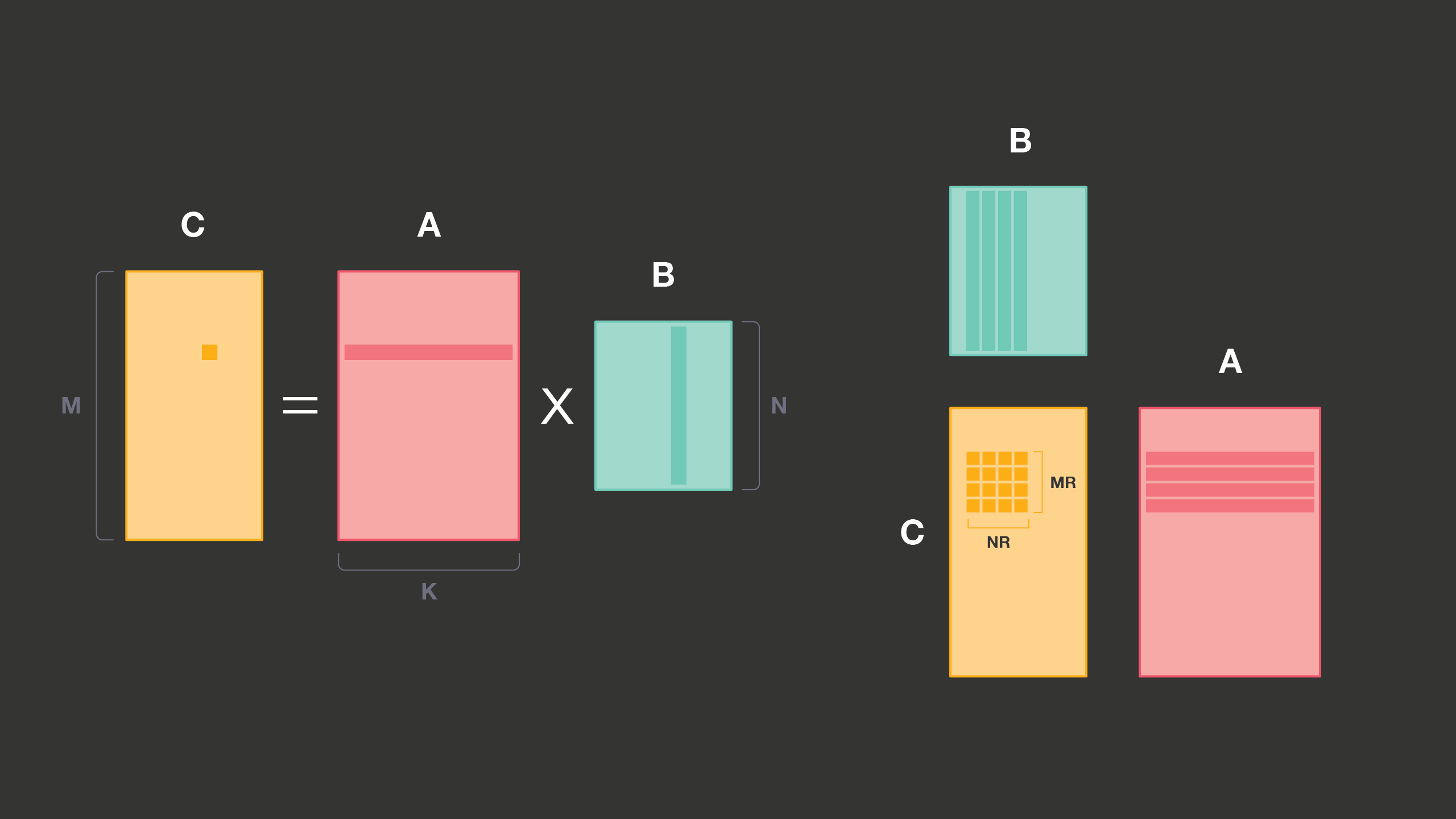Matrix-matrix multiplication of an MxK matrix A and KxN matrix B produces an MxN matrix C. Each element in C can be thought of as a dot product of a corresponding row of A and column of B.

It is possible to implement the whole matrix-matrix multiplication on top of a dot product primitive, but such implementation would be far from efficient. In a dot product, we load two elements per each multiply-add operation, and on modern processors, this implementation would be bottlenecked by memory or cache bandwidth instead of computational power of multiply-add units. But a slight modification — computing dot products of several rows of A and several columns of B simultaneously — dramatically improves performance.

The modified primitive loads MR elements of A and NR elements of B, and performs MRxNR multiply-accumulate operations. The maximum values of MR and NR are limited by the number of registers and other details of processor architecture. But on most modern systems, they are large enough to make the operation compute-bound, and all high-performance matrix-matrix multiplication implementations build upon this primitive, commonly called PDOT (panel dot product) microkernel.

## Quantization in neural networks and how QNNPACK boosts efficiency

PyTorch and other deep learning frameworks commonly use floating-point numbers to represent the weights and neurons of a neural network during training. After model training is finished, though, floating-point numbers and calculations become overkill: Many types of models can be adapted to use low-precision integer arithmetics for inference without noticeable accuracy loss. Low-precision integer representation offers several benefits over single-precision and even half-precision floating point: a 2x-4x smaller memory footprint, which helps keep a neural network model inside small caches of mobile processors; improved performance on memory bandwidth-bound operations; increased energy efficiency; and, on many types of hardware, higher computational throughput.

QNNPACK uses a linear quantization scheme compatible with the Android Neural Networks API. It assumes that quantized values `q[i]` are represented as 8-bit unsigned integers and that they are related to real-valued representations `r[i]` by this formula:

### r[i] = scale * (q[i] – zero_point)

where `scale` is a positive floating-point number, and `zero_point` is an unsigned 8-bit integer, just like `q[i]`.

Although QNNPACK leverages PDOT microkernel, the way other BLAS libraries do, its focus on quantized tensors with 8-bit elements and mobile AI use cases brings a very different perspective to performance optimization. Most BLAS libraries are targeted at scientific computing use cases with matrices as large as thousands of double-precision floating-point elements, but QNNPACK’s input matrices originate from low-precision, mobile-specific computer vision models, and have very different dimensions. In 1×1 convolutions, K is the number of input channels, N is the number of output channels, and M is the number of pixels in the image. On practical mobile-optimized networks, K and N are no greater than 1,024 and are typically in the 32-256 range.

Constraints of mobile architectures dictate that MR and NR do not exceed 8. So even in the biggest models with 1,024 channels, the whole memory block read in the PDOT microkernel is at most 16KB, which fits into level 1 cache even on ultra-low-end mobile cores. This marks an important distinction between QNNPACK and other GEMM implementations: Whereas other libraries repack A and B matrices to better exploit cache hierarchy in hopes of amortizing packing overhead over large number of computations, QNNPACK is optimized for cases when the panels of A and B can fit into L1 cache. So it aims to remove all memory transformations not strictly necessary for computation.In quantized matrix-matrix multiplication, the products of 8-bit integers are typically accumulated into 32-bit intermediate results and then requantized to produce 8-bit outputs. Traditional implementations are optimized for large matrix sizes, when K can be too big to fit panels of A and B into the cache. To make efficient use of cache hierarchy, traditional GEMM implementations split the panels of A and B into fixed-size subpanels along the K dimension, so each panel fits into L1 cache, and then call PDOT microkernel for each subpanel. This cache optimization requires that PDOT microkernels output 32-bit intermediate results, which in the end are added together and requantized to an 8-bit integer.

As QNNPACK is optimized for mobile networks where panels of A and B always fit into L1 cache, it processes the whole panels of A and B in one microkernel call. With no need to accumulate 32-bit intermediate results outside the microkernel, QNNPACK fuses requantization of 32-bit intermediates into the microkernel and writes out 8-bit values, saving memory bandwidth and cache footprint.Fitting the entire panels of A and B into the cache enables another optimization in QNNPACK: eliminated repacking of matrix A. Unlike matrix B, which contains static weights and can be transformed into any memory layout at a one-time cost, matrix A contains convolution input, which changes with every inference run. Thus, repacking matrix A incurs overhead on every run. Despite the overhead, traditional GEMM implementations repack matrix A for two reasons: limited cache associativity and microkernel efficiency. Without repacking, the microkernel would have to read rows of A separated by potentially large stride. If this stride happens to be a multiple of a large power of 2, elements from different rows of A in the panel may fall into the same cache set. If the number of colliding rows exceeds cache associativity, they evict each other and performance falls off a cliff. Fortunately, this situation cannot happen when the panel fits into L1, as with the models for which QNNPACK is optimized.

The effect of packing on microkernel efficiency is closely related to the use of SIMD vector instructions that all modern mobile processors support. These instructions load, store, or compute a small fixed-size vector of elements, rather than a single scalar. It is important to make good use of vector instructions to reach high performance on matrix-matrix multiplication. In a classic GEMM implementation, the microkernel loads repacked MR elements exactly into MR lanes in vector registers. In a QNNPACK implementation, MR elements are not contiguous in memory, and the microkernel has to load them into different vector registers. Increased register pressure forces QNNPACK to use smaller MRxNR tiles, but in practice the difference is small and compensated by elimination of packing overhead. For example, on 32-bit ARM architecture, QNNPACK uses a 4×8 microkernel where 57 percent of vector instructions are multiply-add; gemmlowp library, on the other hand, uses a slightly more efficient 4×12 microkernel where 60 percent of vector instructions are multiply-add.

The microkernel loads several rows of A, multiplies by packed columns of B, accumulates results, and in the end performs requantization and writes out quantized sums. Elements of A and B are quantized as 8-bit integers, but multiplication results are accumulated to 32 bits. Most ARM and ARM64 processors do not have an instruction to perform this operation directly, so it must be decomposed into several supported operations. QNNPACK provides two versions of the microkernel; they differ by the sequence of instructions used to multiply 8-bit values and accumulate them to 32 bits.

## Default microkernel

NEON, the vector extension on ARM architecture, is rich in uncommon instructions. The default microkernel in QNNPACK makes extensive use of two NEON-specific types of instructions: “long” instructions, which produce a vector of elements twice as wide as their inputs; and multiplications of a vector register by an element of another vector register. The microkernel loads vectors of 8-bit unsigned integers, extends them to 16 bits, and uses a vector-by-scalar multiply-add long instruction (`VMLAL.S16` in AArch32 and `SMLAL/SMLAL2` in AArch64) to multiply 16-bit elements with an accumulation to 32 bits.

ARM NEON provides an instruction (`VSUBL.U8` on AArch32 and `USUBL/USUBL2` on AArch64) to subtract vectors of 8-bit integers and produce a vector of 16-bit integer results, and on most ARM microarchitectures, this instruction is just as fast as a simple integer extension instruction (`VMOVL.U8` on AArch32 and `UMOVL/UMOVL2` on AArch64). As an additional optimization, the microkernel combines subtraction of zero point for elements of A and B matrices and extension from 8-bit integers to 16-bit integers.

## Dual-issue microkernel

The default microkernel uses the fewest possible instructions and thus delivers the best performance on low-end cores, which can execute only one NEON instruction per cycle. High-end Cortex-A cores are similarly limited to one NEON integer multiplication instruction per cycle, but can at least partially co-issue NEON integer multiplication and NEON integer addition instructions. It is thus theoretically possible to improve performance by carefully crafting assembly code to execute two instructions in parallel: vector multiply long (`VMULL.U8` in AArch32, `UMULL` in AArch64), to multiply 8-bit elements and produce 16-bit products; and vector pairwise add (`VPADAL.U16` in AArch32, `UADALP` in AArch64), to add together adjacent 16-bit results of multiplication and add them to 32-bit accumulators. Assuming perfect scheduling of vector multiply and vector pairwise add instructions, a dual-issue microkernel could deliver 8 multiply-add results per cycle — twice as many as the default microkernel.

Practical exploitation of dual-issue capability on high-end Cortex-A cores is complicated by several factors. First, dual-issue capability on high-end Cortex-A cores is not perfect and can sustain only a rate of three instructions in two cycles. Second, NEON does not support vector-by-scalar multiplication for 8-bit integer vectors, and we have to use vector-by-vector multiplication with additional instructions (`VEXT.8` on AArch32, `EXT` on AArch64) to rotate vectors of matrix A. Third, as we perform multiplication on 8-bit elements, we can’t subtract zero point before multiplication (the subtraction result is 9 bits wide) and need to precompute sums of rows of A to adjust the accumulated 32-bit result before requantization.

Despite the overhead caused by the factors above, on Cortex-A75 cores, the microkernel that leverages the dual issue capability proves to be 15 percent to 20 percent faster for a sufficiently large channel count (`K > 64`).

## From matrix multiplication to convolution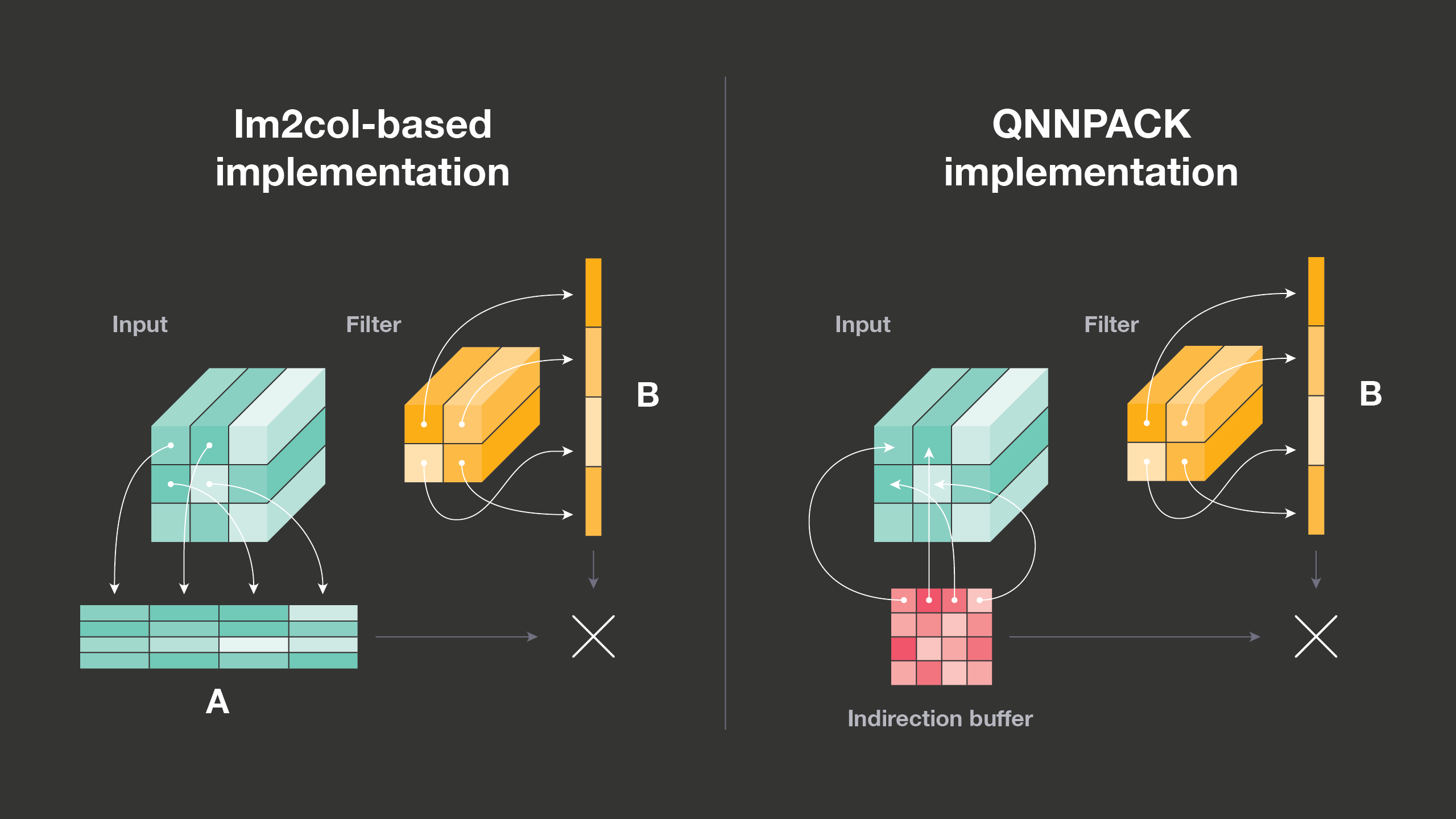Simple 1×1 convolutions directly map to matrix-matrix multiplication, but this is not the case for convolutions with larger kernels, padding, or subsampling (strides). These more sophisticated convolutions, however, can be mapped to matrix-matrix multiplication through a memory transformation called im2col. For each output pixel, im2col copies patches of input image needed to compute it into a 2D matrix. As each output pixel is affected by values of KHxKWxC input pixels, where KH and KW are kernel height and width, and C is the number of channels in the input image, this matrix is KHxKW times larger than the input image, and im2col brings considerable overhead both on memory footprint and performance. Following Caffe, most deep learning frameworks switched to use im2col-based implementation to leverage existing highly optimized matrix-matrix multiplication libraries to do convolution.

In QNNPACK, we implemented a more efficient algorithm. Instead of transforming convolution inputs to fit the implementation of matrix-matrix multiplication, we adapted the implementation of the PDOT microkernel to virtually perform im2col transformation on the fly. Rather than copying actual data from input tensors to the im2col buffer, we set up an indirection buffer with pointers to rows of input pixels that would be involved in computing each output pixel. We also modified the matrix-matrix multiplication microkernel to load pointers to rows of imaginary matrix A from the indirection buffer, which is typically much smaller than the im2col buffer. Moreover, if the memory location of the input tensor doesn’t change between inference runs, the indirection buffer can also be initialized with pointers to rows of input once, and then reused across many inference runs. We observed that the microkernel with indirection buffer not only eliminates the overhead of im2col transformation but also performs slightly better than the matrix-matrix multiplication microkernel (presumably because input rows are reused when computing different output pixels).

## QNNPACK and depthwise convolutions

Grouped convolutions divide input and output channels into several groups and process each group independently. In the limit case, when the number of groups equals the number of channels, the convolution is called depthwise, and it is commonly used in today’s neural network architectures. Depthwise convolution performs spatial filtering of each channel independently and demonstrates a very different computational pattern from normal convolution. For this reason, it is typical to provide a separate implementation for depthwise convolution, and QNNPACK includes a highly optimized version of depthwise 3×3 convolution.

A traditional implementation of depthwise convolution iterates over filter elements one at a time and accumulates the effect of one filter row and one input row on one output row. For a 3×3 depthwise convolution, such implementation would update each output row 9 times. In QNNPACK, we compute the effect of all 3×3 kernel rows and 3×3 input rows on one output row at once, write it out, and then proceed to the next output row.

The key to high performance in QNNPACK implementation is to perfectly utilize general-purpose registers (GPRs) to unroll the loop over filter elements while avoiding reloads for address registers in the hot loop. The 32-bit ARM architecture limits the implementation to only 14 GPRs. In 3×3 depthwise convolution, which is currently one of the most common in mobile-based neural network architecture, we need to read 9 input rows and 9 filter rows. This means we must store 18 addresses if we want to fully unroll the loop. In practice, however, we know the filter is not changing at inference time. So we take filters that were previously in the shape CxKHxKW and pack them into [C/8]xKWxKHx8, so we can use only one GPR with address increment to access all filters. (We use the number 8 because we load 8 elements in one instruction and then subtract zero point, producing eight 16-bit values in a 128-bit NEON register.) We then can fit 9 input row pointers and the pointer to repacked filters in only 10 GPRs and fully unroll the loop over filter elements. The 64-bit ARM architecture doubles the number of GPRs compared with the 32-bit version. QNNPACK leverage the additional ARM64 GPRs to hold pointers to 3×5 input rows and compute 3 output rows at a time.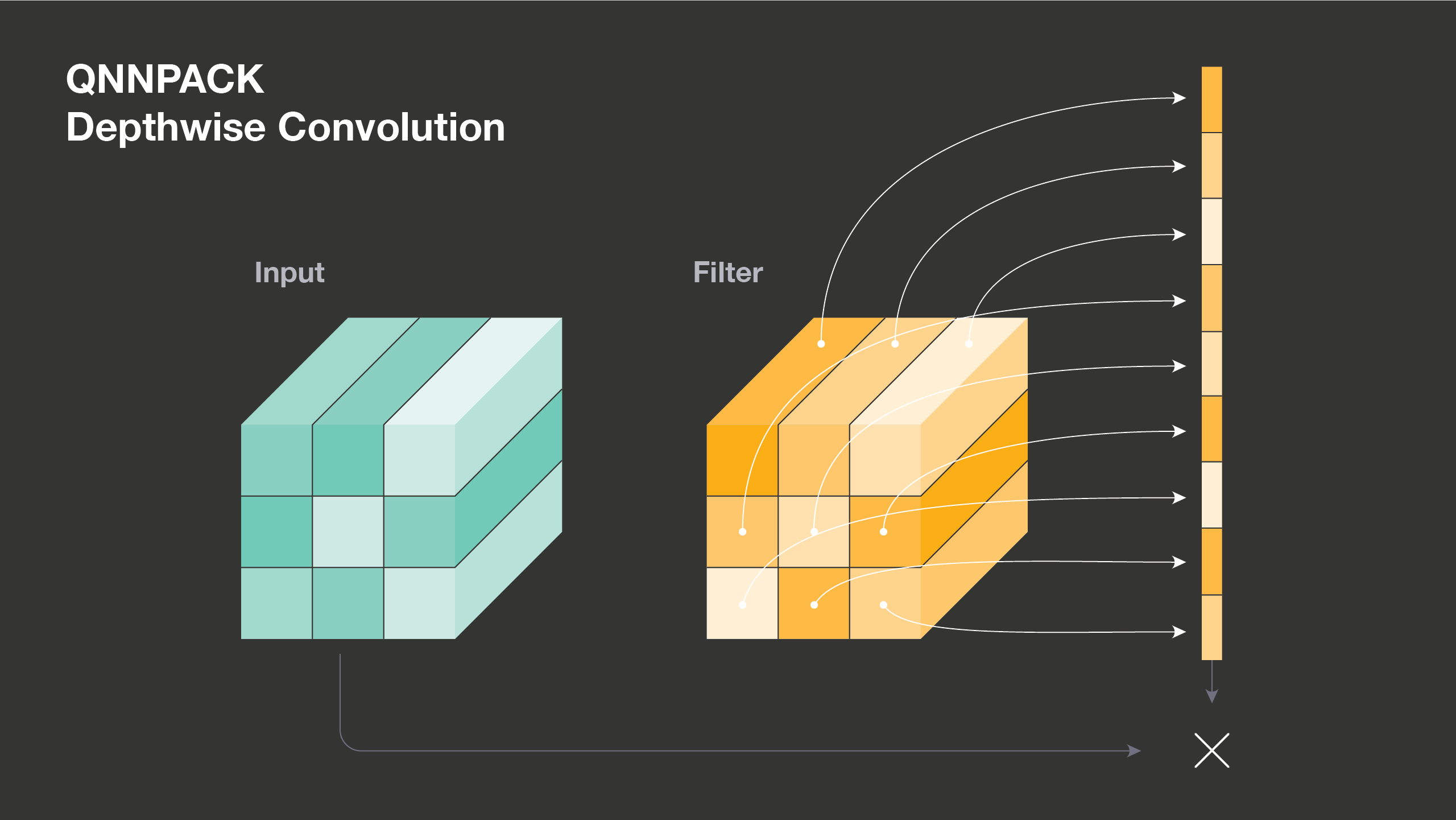Our testing reveals a performance advantage of QNNPACK on end-to-end benchmarks. On quantized state-of-the-art MobileNet v2 architecture, QNNPACK-based Caffe2 operators are approximately 2x faster than TensorFlow Lite on a variety of phones. Together with QNNPACK we are open-sourcing Caffe2 quantized MobileNet v2 model, which achieves 1.3 percent higher top-1 accuracy than the corresponding TensorFlow model.

## MobileNetV1

The first version of the MobileNet architecture pioneered the use of depthwise convolutions to make a model more suitable for mobile devices. MobileNetV1 consists almost entirely of 1×1 convolutions and depthwise 3×3 convolutions. We converted the quantized MobileNetV1 model from TensorFlow Lite and benchmarked it on 32-bit ARM builds of TensorFlow Lite and QNNPACK. With both runtimes using 4 threads, we observed 1.8x geomean speedup of QNNPACK over the TensorFlow Lite runtime.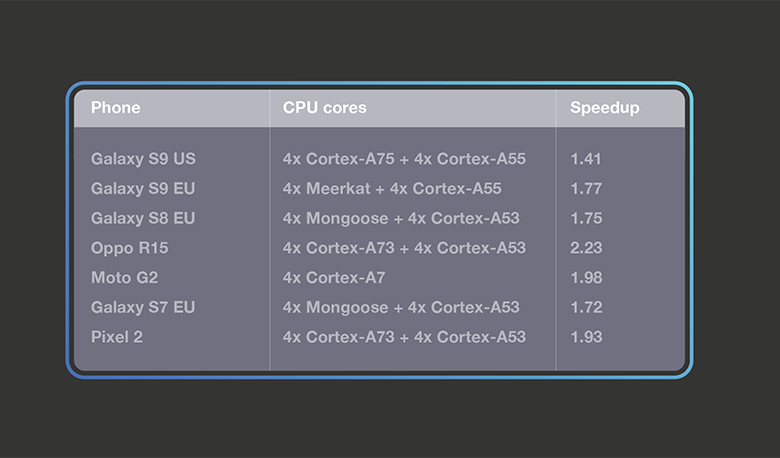### MobileNetV2

As one of the state-of-the-art architectures for mobile visual tasks, MobileNetV2 introduced the bottleneck building block, as well as shortcut connections between bottlenecks. We benchmarked QNNPACK-based Caffe2 operators on a quantized version of the MobileNetV2 classification model against the TensorFlow Lite implementation.We released the quantized Caffe2 MobileNetV2 model here, and used the quantized TensorFlow Lite model from the official repository. The table below shows the top1 accuracy on a commonly used test data set: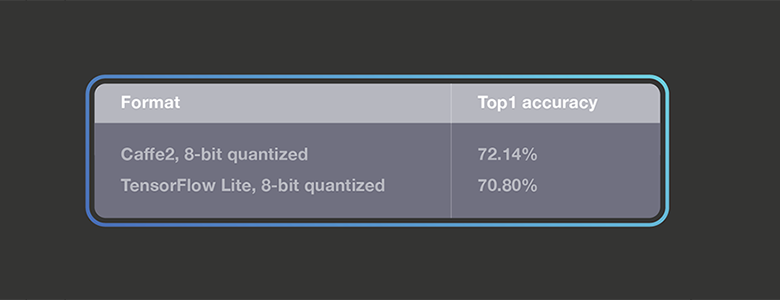Using these models, we set up benchmarks with the Facebook AI Performance Evaluation Platform on a wide range of phones on 32-bit ARM builds. For the TensorFlow Lite thread setup, we tried one to four threads and report the fastest results.We found that TensorFlow Lite performs the best with four threads on these phones, so we used four threads in the benchmarks for both TensorFlow Lite and QNNPACK. The table below presents the results, and demonstrates that on both typical smartphones as well as higher-end phones, QNNPACK-based operators are significantly faster than TensorFlow Lite on MobileNetV2.